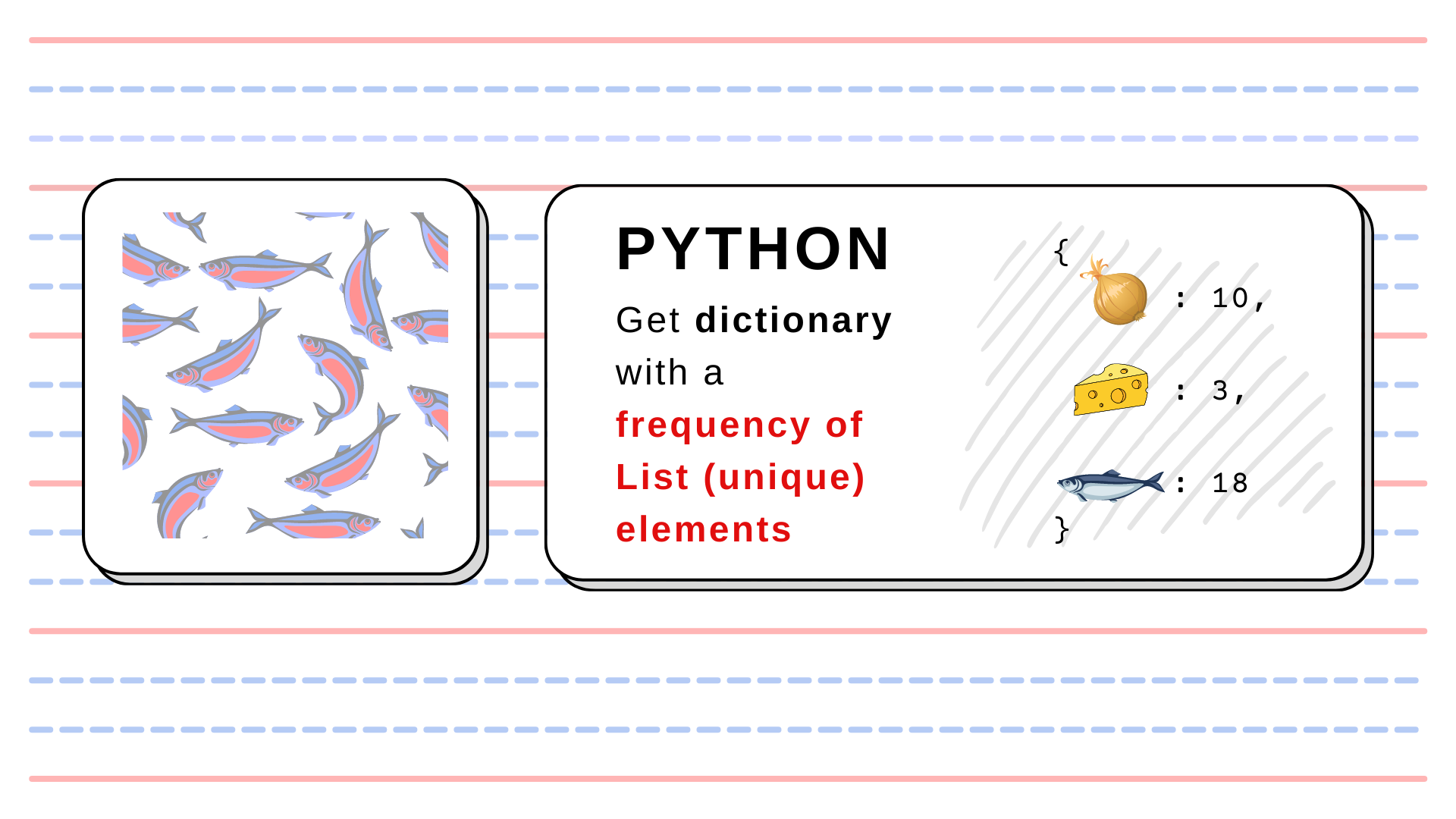Top
Sp.4ML > Data Engineering  > Toolbox: Name and Frequency of unique elements from a List in Python## Toolbox: Name and Frequency of unique elements from a List in Python

Sometimes we need to get names and counts of unique elements from a list or array. Usually, we use pandas method .value_counts() which returns a frequency table. In some cases, it is not convenient to use pandas. Maybe our processing pipeline uses only numpy (a common scenario with ML models), but at some intermediate data processing step, we need to check counts of categorical values. My current use case: I perform clustering and want to log how many values were assigned to each label.

The function for this task is short:

from typing import Dict, Iterable
import numpy as np

def get_categories_frequency(ds: Iterable) -> Dict:
"""
Function calculates how many times each unique category appears in a list.

Parameters
----------
ds : Iterable
List-like object with categorical data.

Returns
-------
: Dict
Dict with {category: frequency} records.
"""
lbls, counts = np.unique(ds, return_counts=True)
zipped = dict(zip(lbls, counts))
return zipped##### Szymon
Subscribe
Notify of